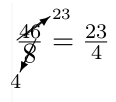LaTeX fractions

StartLaTeX Topics

LaTeX fractions

Fractions belong to the standard applications within mathematics, but they are also occasionally used within normal text.

1.1 LaTeX fraction in math

Fractions are made with the command \frac{numerator}{denominator}. Example for fractions:
 Input Output $\frac{1}{a}$ $\frac{1}{a}$ $\frac{1}{\frac{a}{b}}$ $\frac{1}{\frac{a}{b}}$ $\frac{\frac{a}{b+c}+d}{a +\frac{b}{c+d}}$ $\frac{\frac{a}{b+c}+d}{a +\frac{b}{c+d}}$ $\frac{1}{a}$ $\frac{1}{a}$ $\frac{1}{\frac{a}{b}}$ $\frac{1}{\frac{a}{b}}$ $\frac{\frac{a}{b+c}+d}{a +\frac{b}{c+d}}$ $\frac{\frac{a}{b+c}+d}{a +\frac{b}{c+d}}$

1.1.1 change size of fractions

To display a fraction larger or smaller, the following commands are available from the package amsmath.sty.
 name/command input output displaystyle $\dfrac{1}{\dfrac{a}{b}}$ $\dfrac{1}{\dfrac{a}{b}}$ textstyle $\tfrac{1}{\tfrac{a}{b}}$ $\tfrac{1}{\tfrac{a}{b}}$ scriptstyle ${\frac{1}{\frac{a}{b}} }$ $\frac{1}{\frac{a}{b}}$ scriptscriptstyle ${\scriptscriptstyle \frac{1}{\frac{a}{b}} }$ $\frac{1}{\frac{a}{b}}$

For the latter two sizes scriptstyle and scriptscriptstyle amsmath.sty provides no shortcut commands for these. However, these commands are quite easy to create.

\newcommand{\sfrac}{\genfrac{}{}{}2}
\newcommand{\ssfrac}{\genfrac{}{}{}3}
 $\sfrac{1}{\sfrac{a}{b}}$ $\frac{1}{\frac{a}{b}}$ $\ssfrac{1}{\ssfrac{a}{b}}$ $\scriptscriptstyle \frac{1}{\frac{a}{b}}$

1.1.2 LaTeX continued fraction

Normal continued fraction within $environment:$\frac{1}{1+\frac{1}{1+\frac{1}{1+\frac{1}{1 + \frac{1}{1 + x}}}}}$Output:  $\frac{1}{1+\frac{1}{1+\frac{1}{1+\frac{1}{1 + \frac{1}{1 + x}}}}}$ Normal continued fraction within displaymath environment: $\frac{1}{1+\frac{1}{1+\frac{1}{1+\frac{1}{1 + \frac{1}{1 + x}}}}}$ Output: $\frac{1}{1+\frac{1}{1+\frac{1}{1+\frac{1}{1 + \frac{1}{1 + x}}}}}$ Normal continued fraction by using the \cfrac from amsmath.sty within$ environment:
$\cfrac{1}{1+\cfrac{1}{1+\cfrac{1}{1+\cfrac{1}{1 + \cfrac{1}{1 + x}}}}}$
Output:
 $\cfrac{1}{1+\cfrac{1}{1+\cfrac{1}{1+\cfrac{1}{1 + \cfrac{1}{1 + x}}}}}$

Normal continued fraction by using the \cfrac from amsmath.sty within displaymath environment:
$\cfrac{1}{1+\cfrac{1}{1+\cfrac{1}{1+\cfrac{1}{1 + \cfrac{1}{1 + x}}}}}$

Output:
$\cfrac{1}{1+\cfrac{1}{1+\cfrac{1}{1+\cfrac{1}{1 + \cfrac{1}{1 + x}}}}}$

1.1.3 LaTeX fractions without line or slash

With the command \substack{numerator \\ denominator} you will be able to set a fraction without slash.
$\substack{a\\b}$
 $\substack{a\\b}$

It will also work within displaymath environment:
$\substack{a\\b}$
$\substack{a\\b}$

1.1.4 Parenthesis around fraction

If you want to clamp fractions, you should use the \left and rightvariants.
Without left and right:
$( \frac{a^{2}}{2} )$
Output:
 $( \frac{a^{2}}{2} )$

With left and right:
$\left( \frac{a^{2}}{2} \right)$

Output:
 $\left( \frac{a^{2}}{2} \right)$

LaTeX fractions within text

In principle, a fraction, such as 12 can also be written in normal text, for example with $\tfrac{1}{2}$. Alternatively, there are separate commands for selected fractions, such as for a quarter:\textonequarter{} ¼ and a half: \textonehalf{} ½.

usepackage for fractions

The first package nicefrac.sty serves the better representation in the text. The second package cancel.sty enables the simplifying (reducing) of fractions.

1.3.1 nicefrac usepackage

After you include the package nicefrac the command \nicefrac{numerator}{denominator} is available. And the example sentence looks much nicer, because the fraction is written in the slanting way known for text. In principle, a fraction, such as ½ can also be written in normal text, for example with $\nicefrac{1}{2}$.

1.3.2 cancel usepackage

The cancel.sty usepackage contains the following four commands for simplifying (reducing) of fractions.
 command example description $\cancel{24}$ $\cancel{24}$ Stroke / Line from bottom left to top right $\bcancel{24}$ $\bcancel{24}$ Stroke / Line from top left to bottom right $\xcancel{24}$ $\xcancel{24}$ Two crossing strokes (combination of the first two commands) $\cancelto{23}{46}$ $\cancelto{23}{46}$ Reducing to ...
Input:
\frac{\cancel{24}}{\cancel{8}} = 3$\\ \frac{\cancel{24}}{\bcancel{8}} = 3$\\
\frac{\xcancel{24}}{\bcancel{8}} = 3$\\ \frac{\cancelto{23}{46}}{\cancelto{4}{8}} = \frac{23}{4}$\\
Output:
$\frac{\cancel{24}}{\cancel{8}} = 3$ $\frac{\cancel{24}}{\bcancel{8}} = 3$ $\frac{\xcancel{24}}{\bcancel{8}} = 3$ $\frac{\cancelto{23}{46}}{\cancelto{4}{8}} = \frac{23}{4}$ The first three commands also work with text:
 Input Output \cancel{Text} $\cancel{\text{Text}}$ \bcancel{Text} $\bcancel{\text{Text}}$ \xcancel{Text} $\xcancel{\text{Text}}$
The representation of the cancelto command can be improved a little bit by hand:instead ofTherefore the command cancelto would be changed to:
\newcommand{\bcancelto}{\rotatebox[origin=c]{180}{$\cancelto{\rotatebox[origin=c]{180}{\scriptsize #1}}{#2}$}}
Hint: The command rotatebox is part of the graphicx.sty usepackage, therefore you have to include this usepackage too.

Input:
$\frac{\cancelto{23}{46}}{\bcancelto{4}{8}} = \frac{23}{4} \text{ instead of } \frac{\cancelto{23}{46}}{\cancelto{4}{8}} = \frac{23}{4}$
Output:instead of